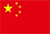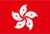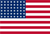Scanning QR Code• Online Inquiry

+
Congratulations
You've successfully got advanced quotes worth 5000 HKD
• Home
• Quotes
• News
• Community
• Learn
• Help Center
• Language
•••EN

# Greeks

The price of an option influenced by the price of underlying asset, the expected volatility of the underlying stock's price, option strike price, maturity and interest rate etc. Greeks are usually used to indicate the sensitivity of the option price to changes in the above-mentioned influencing factors. Those measures are important in managing risk of options. 「Greeks」 which are used in options pricing are shown in the following table:

 Greek symbol description Delta Δ the rate of change between the option's price and the underlying asset's price Gamma γ the rate of change between an option portfolio's delta and the underlying asset's price Theta θ the rate of decline in the value of an option due to the passage of time. Vega ν the rate of change between the option's price and the implied volatility. Rho P Option price sensitivity to risk-free interest rate changes.

Delta

Delta，referred to as the "hedge ratio", represents the rate of change between the option's price and the underlying asset's price - in other words, price sensitivity. The ratio comparing the change in the price of the underlying asset to the corresponding change in the price of a derivative.

Delta could be used in two ways：

1. Delta Hedging

Assume one holds 1 option and writes delta shares of ETF, according to the hedge ratio, a \$0.001 increase in stock price will result in a loss of \$Delta on the stock holdings, whereas the gain on the 1 option written will be 0.001*\$Delta. The stock price movement leaves total wealth unaltered, which is what hedged position is intended to do.

Calculating leverage

For example, if the price of ETF rises 1% and the option rises by 10%, then the leverage of the option is 10 times. Through Delta, we can calculate the leverage of the option. Assuming the ETF price is \$3.000, a one-month call option with an exercise price of 3.20, the current price is \$0.1000, and the Delta is 0.33. If the ETF rises 1%, which is \$0.030, then the option price will rise 0.030*Delta, which is equal to \$0.01. From the perspective of the change, the option contract has risen 10%. Therefore, the leverage of an option contract is approximately 10 times

Gamma

Gamma represents the rate of change between an option portfolio's delta and the underlying asset's price - in other words, second-order time price sensitivity. For example, the delta changes 0.001*Gamma with respect to the price of the ETF changes \$0.001.

Suppose one holds 1 option and writes Delta shares of ETF, Delta changes as the price of the ETF changes. When the price of the ETF changes, the position of the ETF, Delta, should be adjusted in order to hedge the risk. A \$0.001 changes in stock price will result in 0.001*Gamma changes on the ETF holdings. In a word, gamma indicated the difficulties of the hedge.

Theta

Theta represents the rate of change between an option portfolio and time, or time sensitivity. If everything is held constant, then the option will lose value as time moves closer to the maturity of the option.

Vega

Generally, the greater the uncertainty, the higher the risk, and the one who bears the risk requires higher compensation. As for options, expected volatility refers to the amount of uncertainty or risk about the size of changes in a security's value. Similar to premiums, option sellers will also charge higher option fees for options corresponding to assets with relatively large expected volatility.

Vega represents the rate of change between an option portfolio's value and the underlying asset's volatility. If everything is held constant, the price of the option will rise as the expected volatility of the underlying asset rise. Therefore, regardless of Call or Put option, Vega is greater than zero.

Rho

Rho represents the rate of change between an option portfolio's value and the interest rate, or sensitivity to the interest rate. It expresses the change in the option price for every 1% change in interest rate. The higher underlying asset price, the longer the maturity, the larger the Rho. The Rho of the call option is positive, and the put is negative. Compared to other Greeks, Rho has the smallest influence on the option.

*All the data and information above are for reference only and do not constitute any investment advice. Neither Futu or any Market Data Provides shall be directly or indirectly liable for any damage whatsoever resulting directly or indirectly from the content, reliability, integrity, comprehensiveness, accuracy or quality of the Market Data. Futu shall not be directly or indirectly liable for any damages whatsoever, including consequential loss, special, indirect or punitive damages, resulting from or arising out of an interruption or of the transmission of the Market Data.

Back to the Top